# ARRAYS Numerical Arrays of Multiple Dimensions Arrays n

• Slides: 44
Download presentation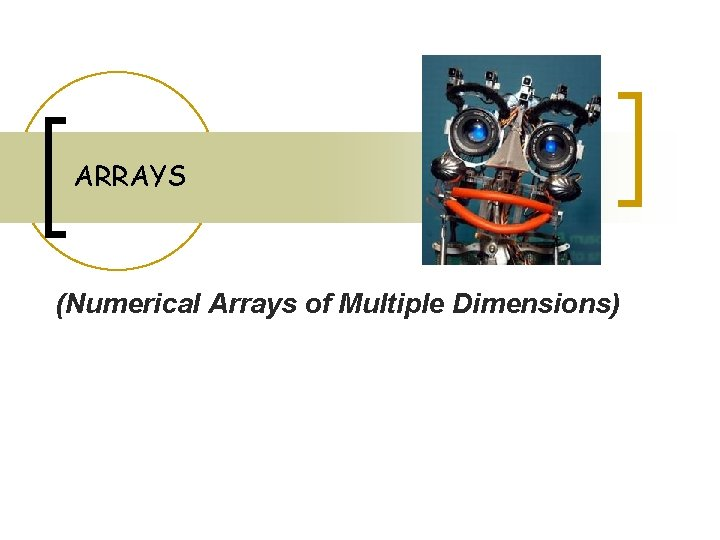ARRAYS (Numerical Arrays of Multiple Dimensions)Arrays n n n We study arrays of more than one dimension: These data structures are widely used in software to model tables of data, matrices, maps, relationship among the variables of a system and images of all kinds-photographic, radar, X- ray, ultrasound, infrared, and magnetic resonance. We show to declare and reference multidimensional arrays, that is , array with two or more dimensions.Array n Arrays of arrays are also called two-dimensional arrays. n If it is possible with strings, it is also possible with numerical arrays.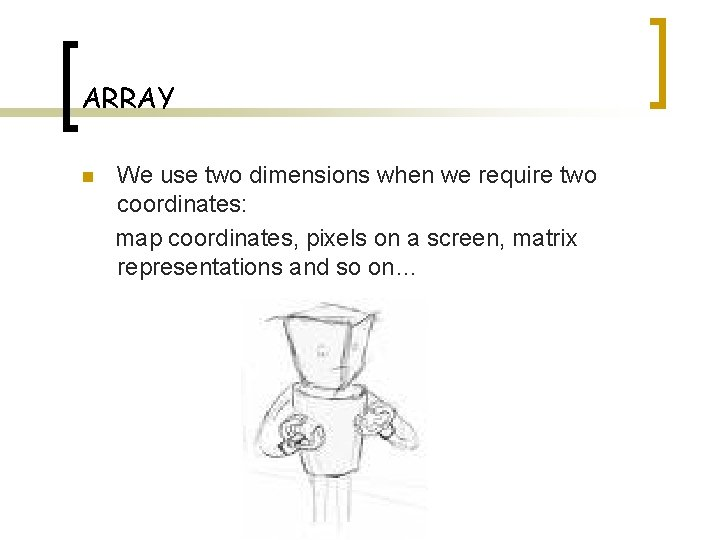ARRAY n We use two dimensions when we require two coordinates: map coordinates, pixels on a screen, matrix representations and so on…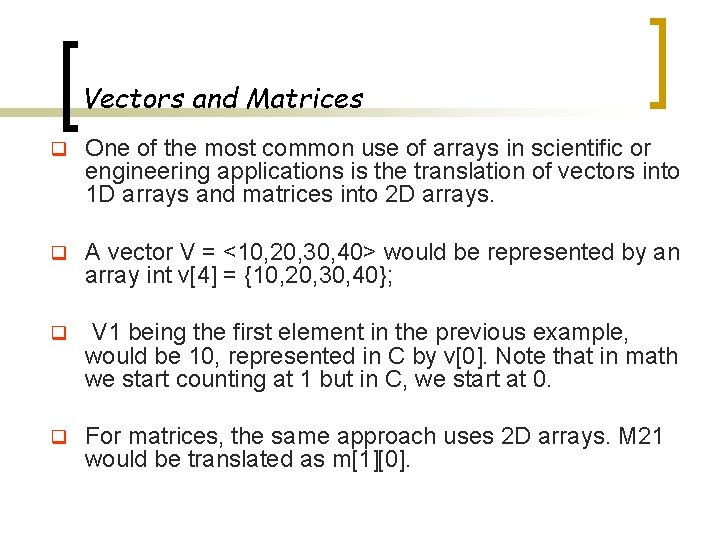Vectors and Matrices q One of the most common use of arrays in scientific or engineering applications is the translation of vectors into 1 D arrays and matrices into 2 D arrays. q A vector V = <10, 20, 30, 40> would be represented by an array int v = {10, 20, 30, 40}; q V 1 being the first element in the previous example, would be 10, represented in C by v. Note that in math we start counting at 1 but in C, we start at 0. q For matrices, the same approach uses 2 D arrays. M 21 would be translated as m.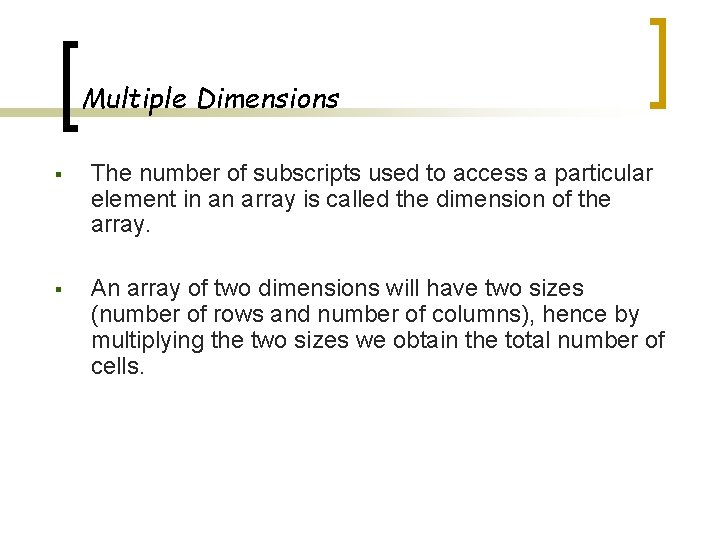Multiple Dimensions § The number of subscripts used to access a particular element in an array is called the dimension of the array. § An array of two dimensions will have two sizes (number of rows and number of columns), hence by multiplying the two sizes we obtain the total number of cells.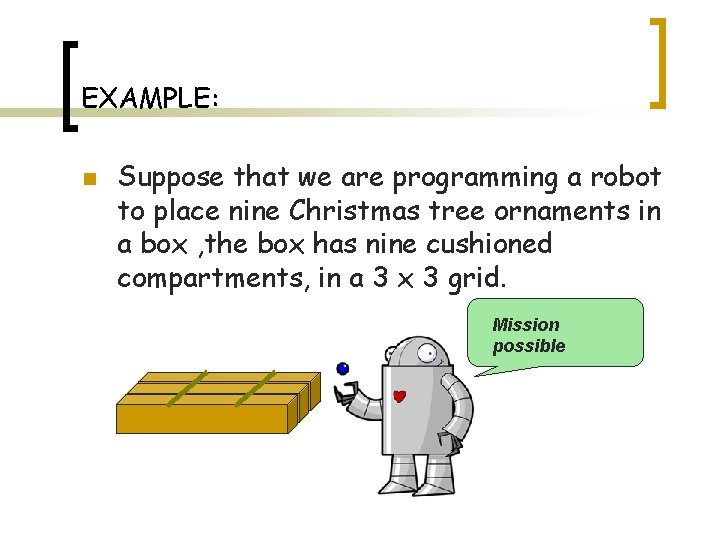EXAMPLE: n Suppose that we are programming a robot to place nine Christmas tree ornaments in a box , the box has nine cushioned compartments, in a 3 x 3 grid. Mission possible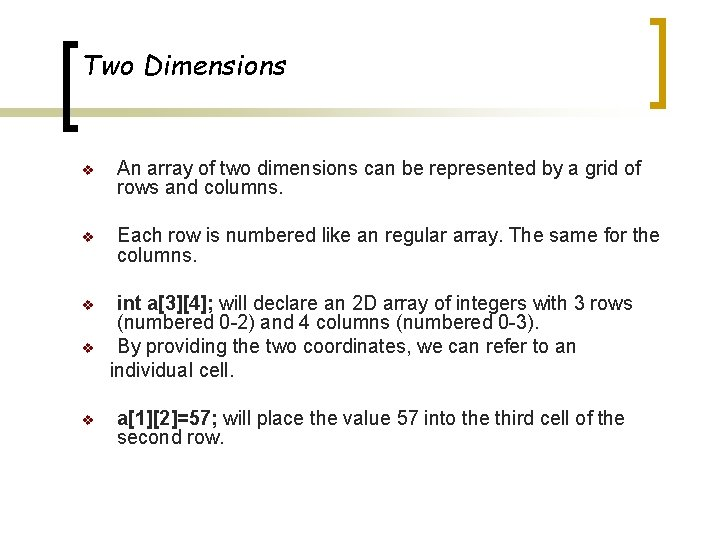Two Dimensions v An array of two dimensions can be represented by a grid of rows and columns. v Each row is numbered like an regular array. The same for the columns. v v v int a; will declare an 2 D array of integers with 3 rows (numbered 0 -2) and 4 columns (numbered 0 -3). By providing the two coordinates, we can refer to an individual cell. a=57; will place the value 57 into the third cell of the second row.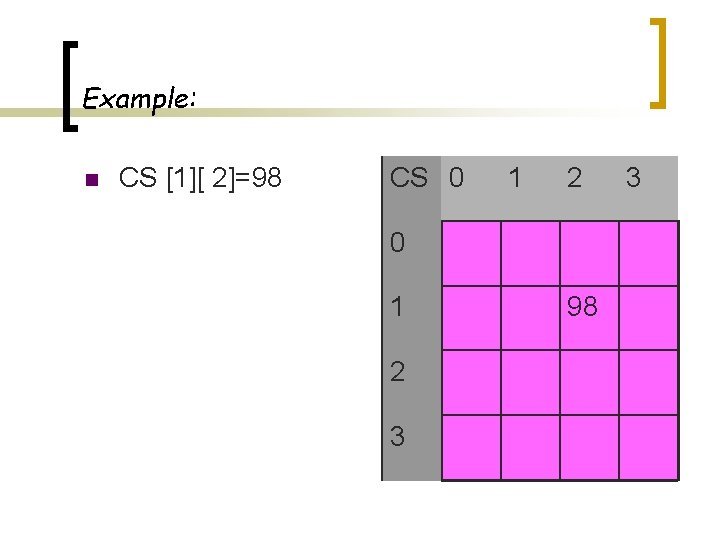Example: n CS [ 2]=98 CS 0 1 2 3 98 3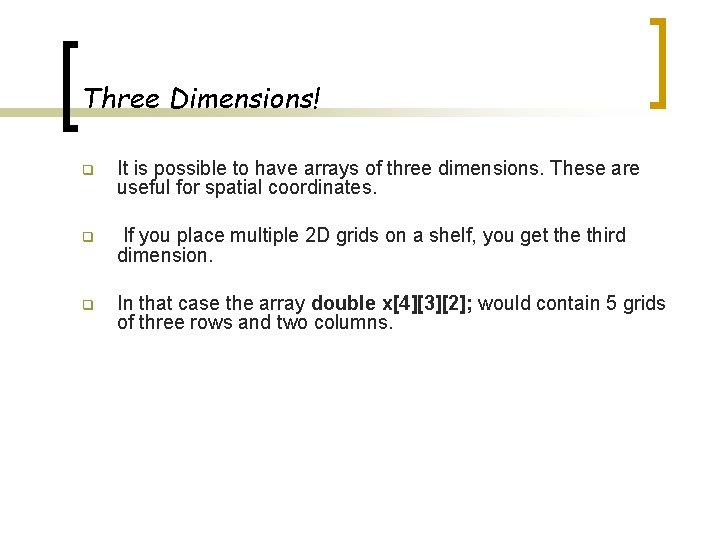Three Dimensions! q It is possible to have arrays of three dimensions. These are useful for spatial coordinates. q If you place multiple 2 D grids on a shelf, you get the third dimension. q In that case the array double x; would contain 5 grids of three rows and two columns.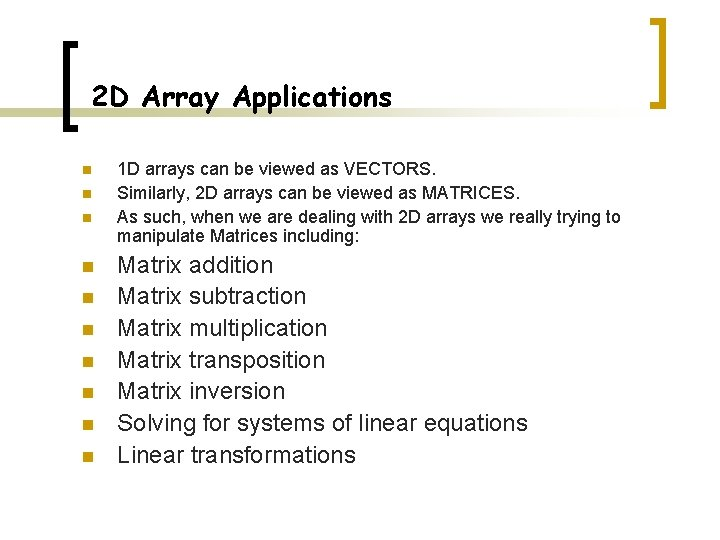2 D Array Applications n n n n n 1 D arrays can be viewed as VECTORS. Similarly, 2 D arrays can be viewed as MATRICES. As such, when we are dealing with 2 D arrays we really trying to manipulate Matrices including: Matrix addition Matrix subtraction Matrix multiplication Matrix transposition Matrix inversion Solving for systems of linear equations Linear transformations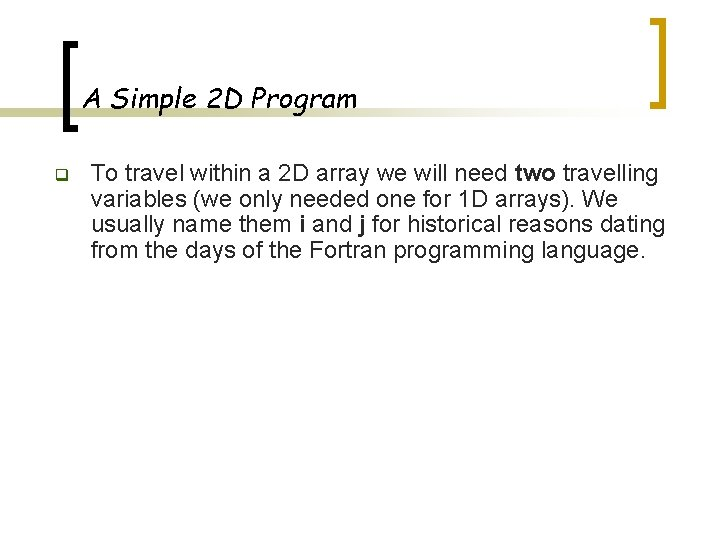A Simple 2 D Program q To travel within a 2 D array we will need two travelling variables (we only needed one for 1 D arrays). We usually name them i and j for historical reasons dating from the days of the Fortran programming language.How to Decalre and Initialize a 2 D Array? n /* PROGRAM # 80 */ n /* INITIALIZE A 2 D ARRAY TO NON-ZERO */ #include <stdio. h> #define row 3 #define column 3 main( ){ int i, j; /*Declare and initialize a 2 D array */ int List[row][column] = { {2, 4, 6}, {8, 10, 12}, {14, 16, 18} }; n n n /* Find out addresses */ for(i=0; i< row; i++){ for(j=0; j< column; j++) printf("%5 d", &List[i][j]); n puts(“”); n n n } /* Print the matrix */ for(i=0; i< row; i++){ for(j=0; j< column; j++) printf("%3 d", List[i][j]); n puts(“”); n n }}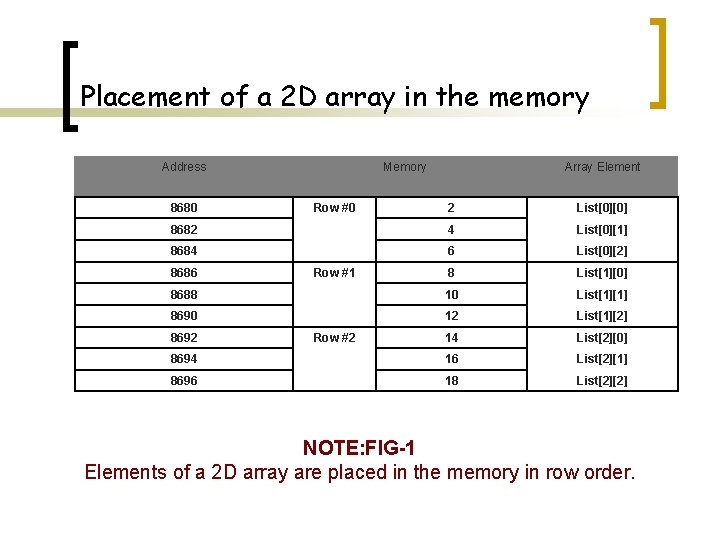Placement of a 2 D array in the memory Address 8680 Memory 2 List 8682 4 List 8684 6 List 8 List 8688 10 List 8690 12 List 14 List 8694 16 List 8696 18 List 8686 8692 Row #0 Array Element Row #1 Row #2 NOTE: FIG-1 Elements of a 2 D array are placed in the memory in row order.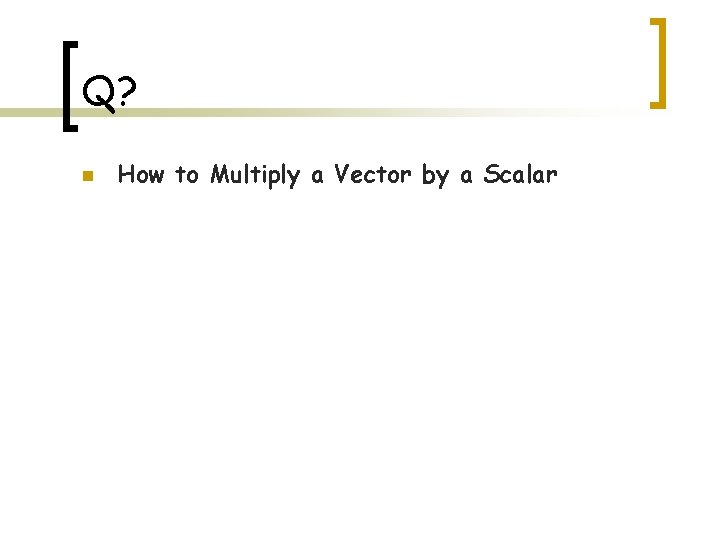Q? n How to Multiply a Vector by a Scalar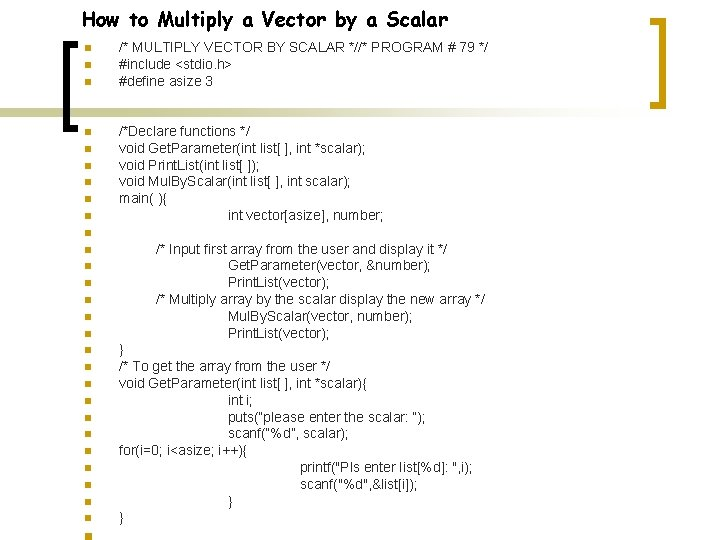How to Multiply a Vector by a Scalar n n n n n /* MULTIPLY VECTOR BY SCALAR *//* PROGRAM # 79 */ #include <stdio. h> #define asize 3 /*Declare functions */ void Get. Parameter(int list[ ], int *scalar); void Print. List(int list[ ]); void Mul. By. Scalar(int list[ ], int scalar); main( ){ int vector[asize], number; n n n n n /* Input first array from the user and display it */ Get. Parameter(vector, &number); Print. List(vector); /* Multiply array by the scalar display the new array */ Mul. By. Scalar(vector, number); Print. List(vector); } /* To get the array from the user */ void Get. Parameter(int list[ ], int *scalar){ int i; puts(“please enter the scalar: “); scanf(“%d”, scalar); for(i=0; i<asize; i++){ printf("Pls enter list[%d]: ", i); scanf("%d", &list[i]); } }/* PROGRAM # 79 n n n */… /* To multiply a vector by a scalar*/ void Mul. By. Scalar(int list[ ], int scalar){ int i; for(i=0; i<asize; i++) list[i] *= scalar; n n n n n } /* To printout the elements of the array */ void Print. List(int list[ ]){ int i; for(i=0; i<asize; i++) printf("list[%d] is %dn", i, list[i]); puts(“”); }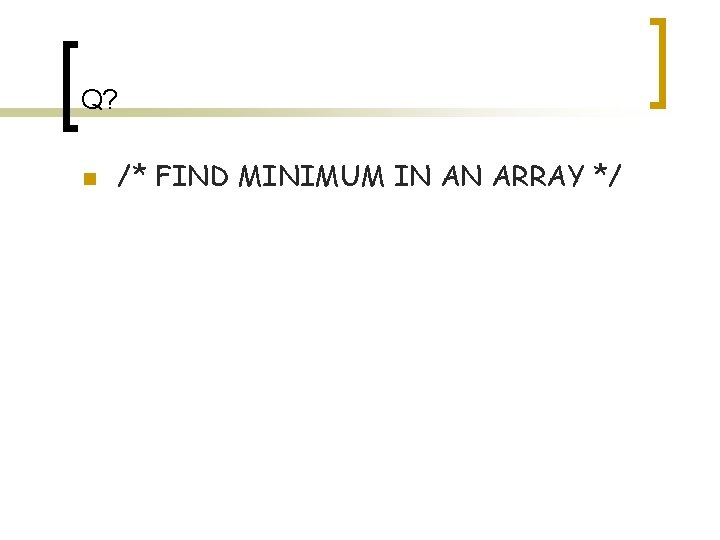Q? n /* FIND MINIMUM IN AN ARRAY */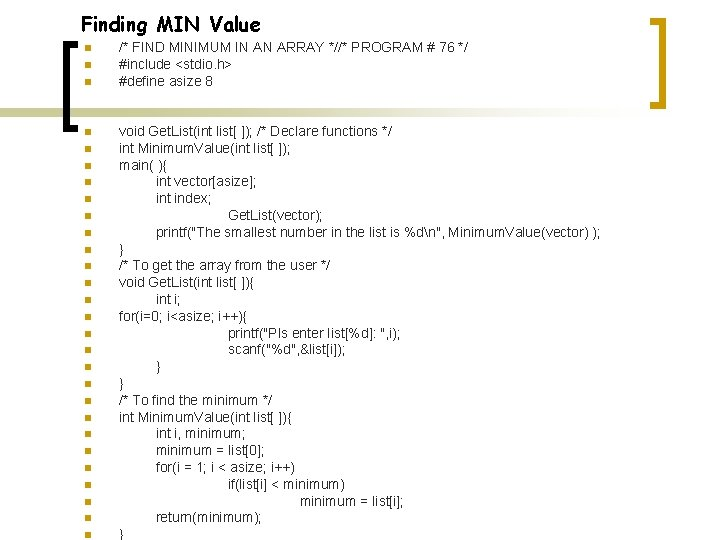Finding MIN Value n n n n n n n /* FIND MINIMUM IN AN ARRAY *//* PROGRAM # 76 */ #include <stdio. h> #define asize 8 void Get. List(int list[ ]); /* Declare functions */ int Minimum. Value(int list[ ]); main( ){ int vector[asize]; int index; Get. List(vector); printf("The smallest number in the list is %dn", Minimum. Value(vector) ); } /* To get the array from the user */ void Get. List(int list[ ]){ int i; for(i=0; i<asize; i++){ printf("Pls enter list[%d]: ", i); scanf("%d", &list[i]); } } /* To find the minimum */ int Minimum. Value(int list[ ]){ int i, minimum; minimum = list; for(i = 1; i < asize; i++) if(list[i] < minimum) minimum = list[i]; return(minimum); }Q? n n /* ADD UP TWO ARRAYS */ /* TWO ARRAYS MUST BE OF THE SAME SIZE */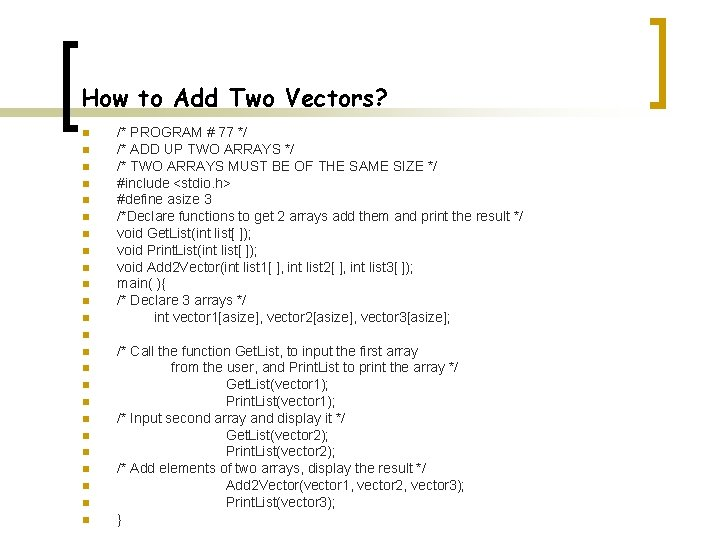How to Add Two Vectors? n n n /* PROGRAM # 77 */ /* ADD UP TWO ARRAYS */ /* TWO ARRAYS MUST BE OF THE SAME SIZE */ #include <stdio. h> #define asize 3 /*Declare functions to get 2 arrays add them and print the result */ void Get. List(int list[ ]); void Print. List(int list[ ]); void Add 2 Vector(int list 1[ ], int list 2[ ], int list 3[ ]); main( ){ /* Declare 3 arrays */ int vector 1[asize], vector 2[asize], vector 3[asize]; n n n /* Call the function Get. List, to input the first array from the user, and Print. List to print the array */ Get. List(vector 1); Print. List(vector 1); /* Input second array and display it */ Get. List(vector 2); Print. List(vector 2); /* Add elements of two arrays, display the result */ Add 2 Vector(vector 1, vector 2, vector 3); Print. List(vector 3); }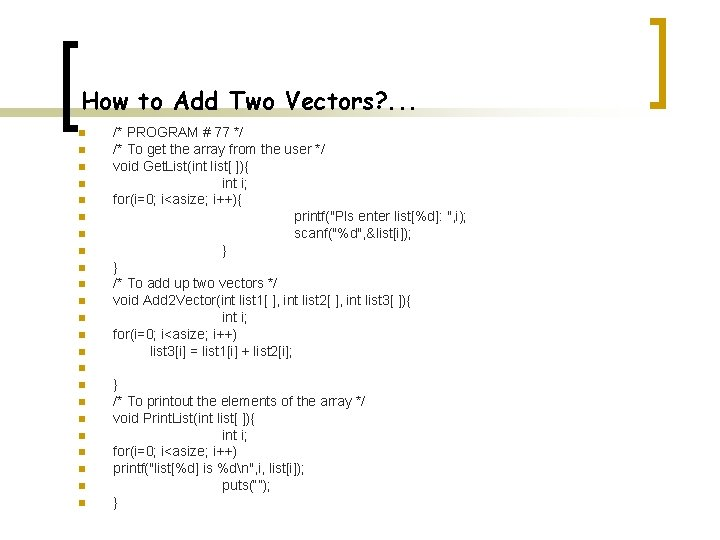How to Add Two Vectors? . . . n n n n /* PROGRAM # 77 */ /* To get the array from the user */ void Get. List(int list[ ]){ int i; for(i=0; i<asize; i++){ printf("Pls enter list[%d]: ", i); scanf("%d", &list[i]); } } /* To add up two vectors */ void Add 2 Vector(int list 1[ ], int list 2[ ], int list 3[ ]){ int i; for(i=0; i<asize; i++) list 3[i] = list 1[i] + list 2[i]; n n n n n } /* To printout the elements of the array */ void Print. List(int list[ ]){ int i; for(i=0; i<asize; i++) printf("list[%d] is %dn", i, list[i]); puts(“”); }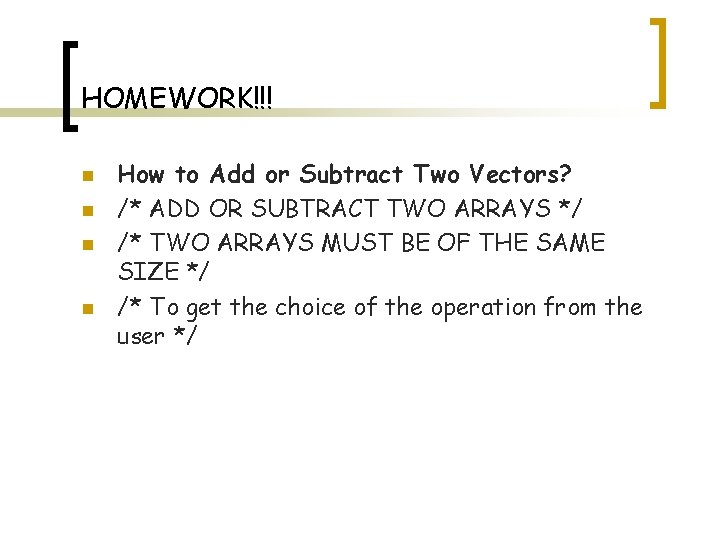HOMEWORK!!! n n How to Add or Subtract Two Vectors? /* ADD OR SUBTRACT TWO ARRAYS */ /* TWO ARRAYS MUST BE OF THE SAME SIZE */ /* To get the choice of the operation from the user */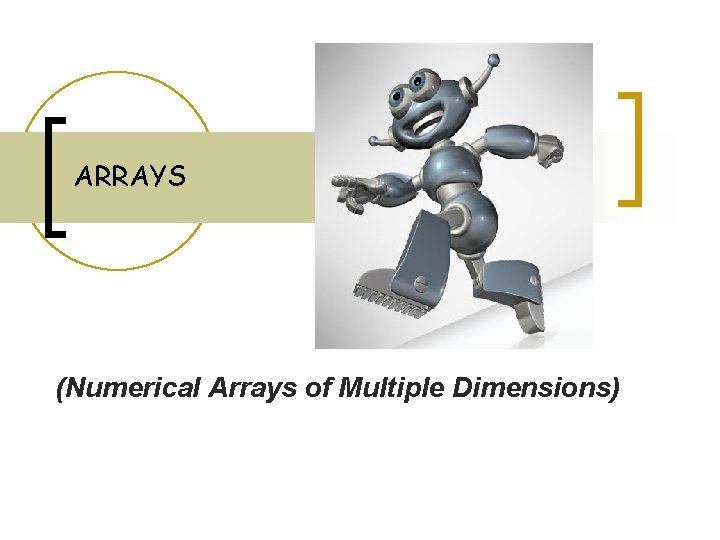ARRAYS (Numerical Arrays of Multiple Dimensions)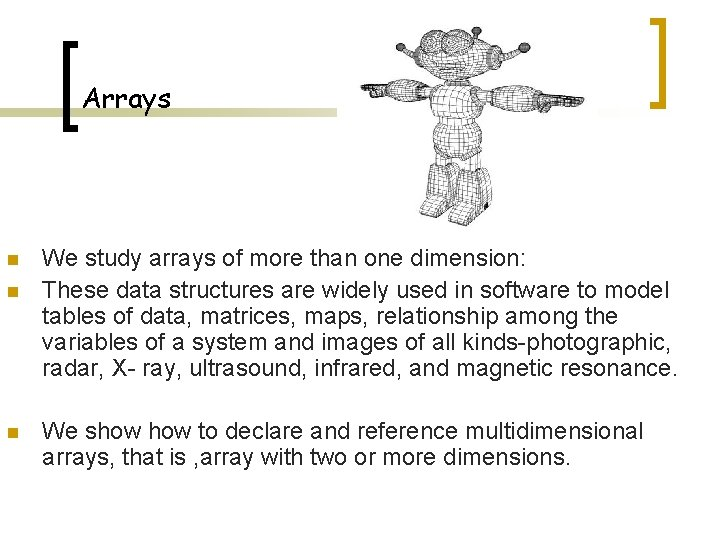Arrays n n n We study arrays of more than one dimension: These data structures are widely used in software to model tables of data, matrices, maps, relationship among the variables of a system and images of all kinds-photographic, radar, X- ray, ultrasound, infrared, and magnetic resonance. We show to declare and reference multidimensional arrays, that is , array with two or more dimensions.Traversing the Elements of a 2 D Array /* 3 METHODS OF MANIPULATING OF ELEMENTS OF A MATRIX*/ n n n n n /* PROGRAM #80 -B */ /* 3 METHODS OF MANIPULATING OF ELEMENTS OF A MATRIX*/ #include<stdio. h> #define row 3 #define column 3 main( ){ int Matrix[row][column]={ {1, 3, 5}, {7, 9, 11}, {13, 15, 17} }; int i, j, *ptr; int *First. Element=&Matrix; int *Last. Element=&Matrix[row-1][column-1]; n n n }/* METHOD 1 */ Ø Ø Ø Ø /* Use of two FOR loops, the outer loop for moving along rows, */ /* the inner loop for moving along columns */ for(i = 0; i < row; i++) for( j = 0; j < column; j++) printf(“%5 d”, Matrix[i][j]); puts(“”);/* METHOD 2 */ q q q /* PTR is a pointer pointing to the 1 st element of the matrix. This pointer gets incremented by one through the For loop */ for(ptr = First. Element; ptr <= Last. Element; ptr++) printf(“%5 d”, *ptr);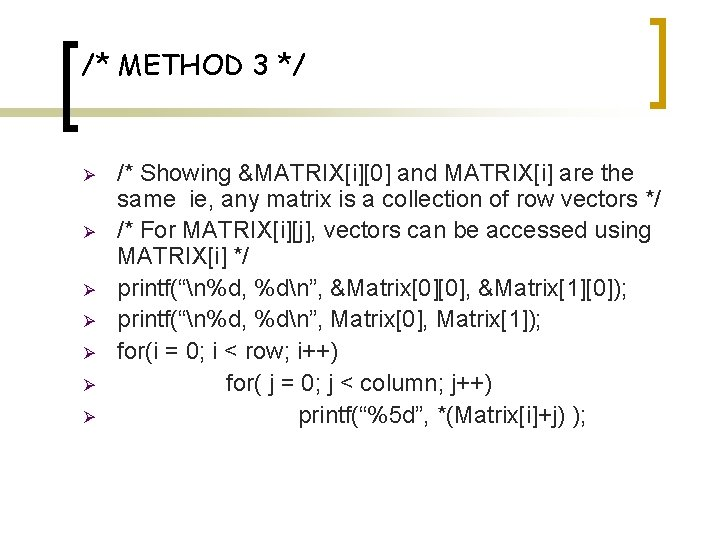/* METHOD 3 */ Ø Ø Ø Ø /* Showing &MATRIX[i] and MATRIX[i] are the same ie, any matrix is a collection of row vectors */ /* For MATRIX[i][j], vectors can be accessed using MATRIX[i] */ printf(“n%d, %dn”, &Matrix, &Matrix); printf(“n%d, %dn”, Matrix, Matrix); for(i = 0; i < row; i++) for( j = 0; j < column; j++) printf(“%5 d”, *(Matrix[i]+j) );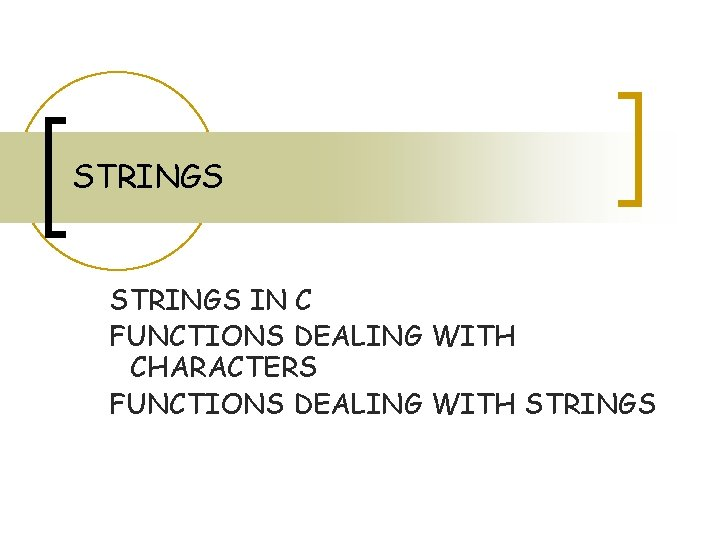STRINGS IN C FUNCTIONS DEALING WITH CHARACTERS FUNCTIONS DEALING WITH STRINGSStrings q q There is no string type in C. Instead, to approximate strings, we will use arrays of characters. To transform a simple array of characters into a string, it must contain the '‘ character in the last cell. Note that '' is one character. It is called the null character or the end-of-string character.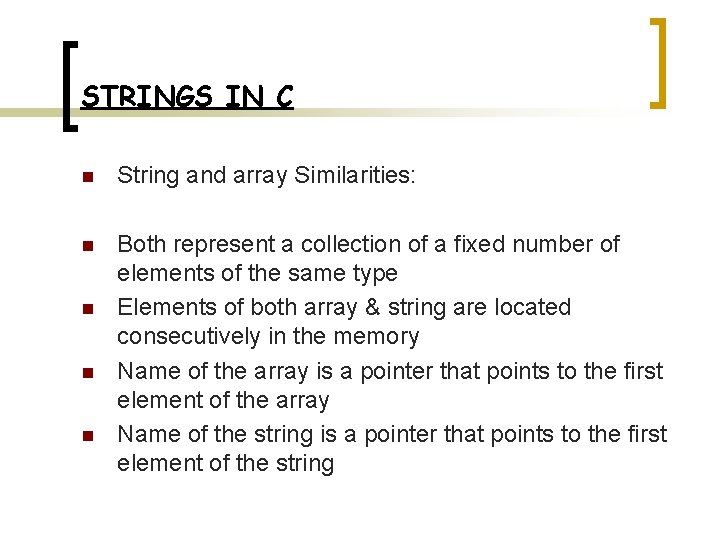STRINGS IN C n String and array Similarities: n Both represent a collection of a fixed number of elements of the same type Elements of both array & string are located consecutively in the memory Name of the array is a pointer that points to the first element of the array Name of the string is a pointer that points to the first element of the string n n nSTRINGS IN C n String and array Differences: n Array represents a collection of numerical values, Data types include int, float, double n n n String represents a collection of characters Data type include only characters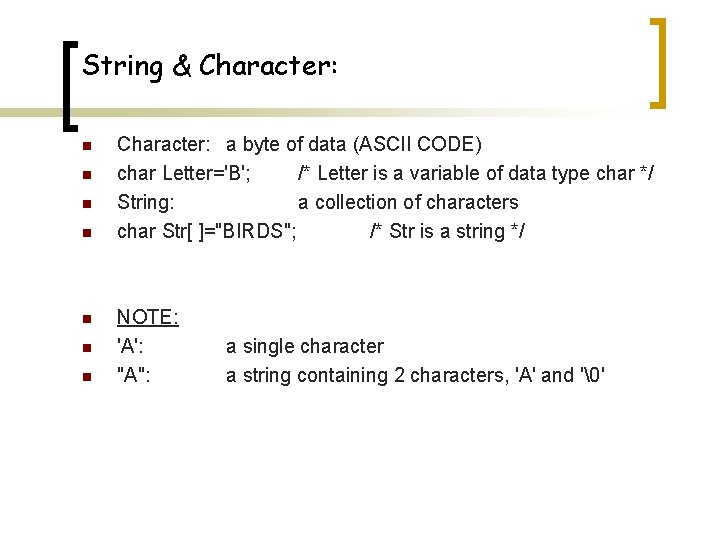String & Character: n n n n Character: a byte of data (ASCII CODE) char Letter='B'; /* Letter is a variable of data type char */ String: a collection of characters char Str[ ]="BIRDS"; /* Str is a string */ NOTE: 'A': "A": a single character a string containing 2 characters, 'A' and ''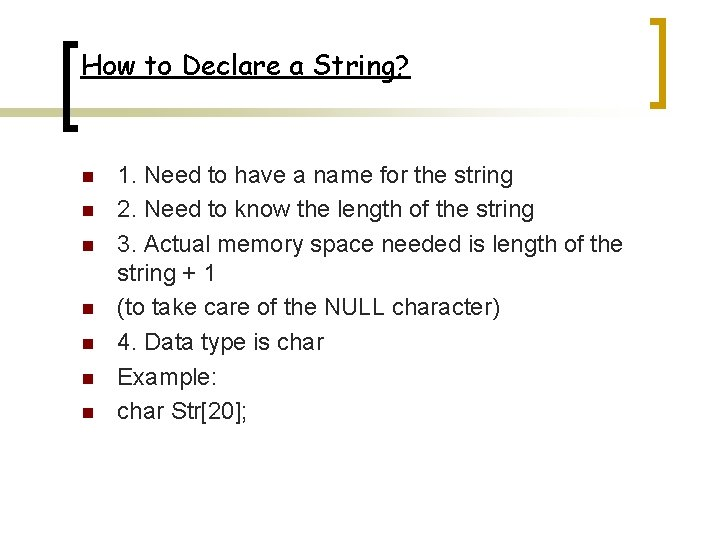How to Declare a String? n n n n 1. Need to have a name for the string 2. Need to know the length of the string 3. Actual memory space needed is length of the string + 1 (to take care of the NULL character) 4. Data type is char Example: char Str;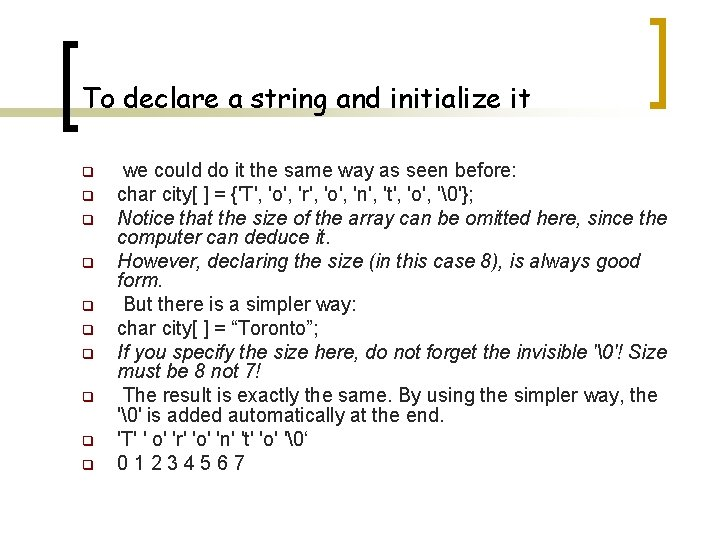To declare a string and initialize it q q q q q we could do it the same way as seen before: char city[ ] = {'T', 'o', 'r', 'o', 'n', 't', 'o', ''}; Notice that the size of the array can be omitted here, since the computer can deduce it. However, declaring the size (in this case 8), is always good form. But there is a simpler way: char city[ ] = “Toronto”; If you specify the size here, do not forget the invisible ''! Size must be 8 not 7! The result is exactly the same. By using the simpler way, the '' is added automatically at the end. 'T' ' o' 'r' 'o' 'n' 't' 'o' '‘ 01234567“U" and ‘U' q Double quotes " " represent a string, A single quote ' ', one character. q ‘U' is the single character U But “U" is an array size 2 containing the ‘U' character and the '' character. q All string constants are represented in double quotes (remember "This is my first C program. " ? ).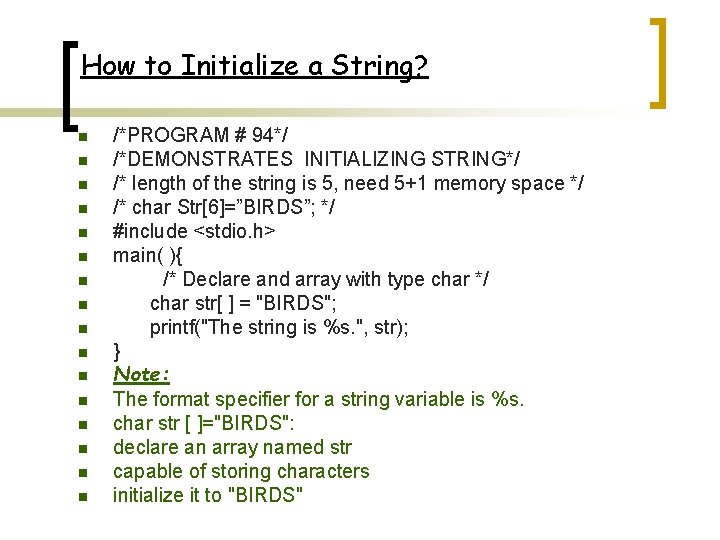How to Initialize a String? n n n n /*PROGRAM # 94*/ /*DEMONSTRATES INITIALIZING STRING*/ /* length of the string is 5, need 5+1 memory space */ /* char Str=”BIRDS”; */ #include <stdio. h> main( ){ /* Declare and array with type char */ char str[ ] = "BIRDS"; printf("The string is %s. ", str); } Note: The format specifier for a string variable is %s. char str [ ]="BIRDS": declare an array named str capable of storing characters initialize it to "BIRDS"Inside the Memory q q q char Str=”BIRDS”; char Str[ ]=”BIRDS”; These 2 statements are equal.Memory Map – Placement of a string within the memory Address memory Array element 2400 ‘B’ Str 2401 ‘I’ Str 2402 ‘R’ Str 2403 ‘D’ Str 2404 ‘S’ Str 2405 ‘’ StrNOTES!!! q Str and &str both refer to the top of the string (ie, address 2400). q ‘’ is NULL character. q ‘’ lets ‘C’ knows where the string ends. q When we calculate the length of the string, '' is NOT counted. q The length of the string is 5 but we need 6 bytes for the string (one extra for ‘’). q Therefore, when declaring the string we need (Length. Of. String+1) bytes.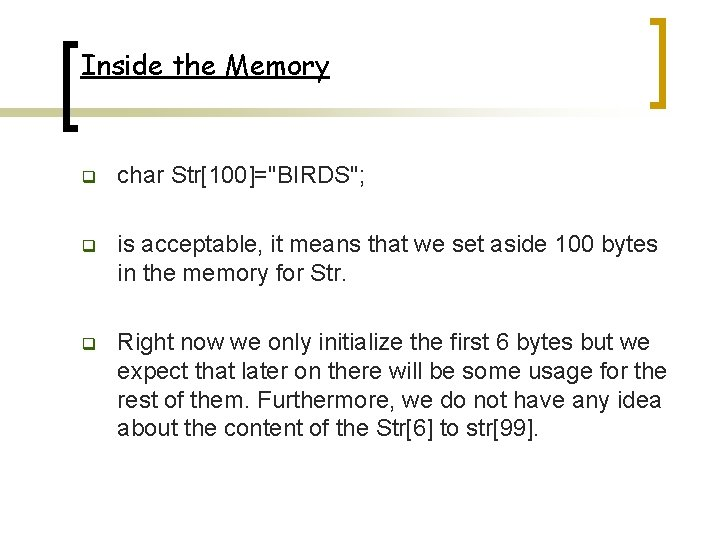Inside the Memory q char Str="BIRDS"; q is acceptable, it means that we set aside 100 bytes in the memory for Str. q Right now we only initialize the first 6 bytes but we expect that later on there will be some usage for the rest of them. Furthermore, we do not have any idea about the content of the Str to str.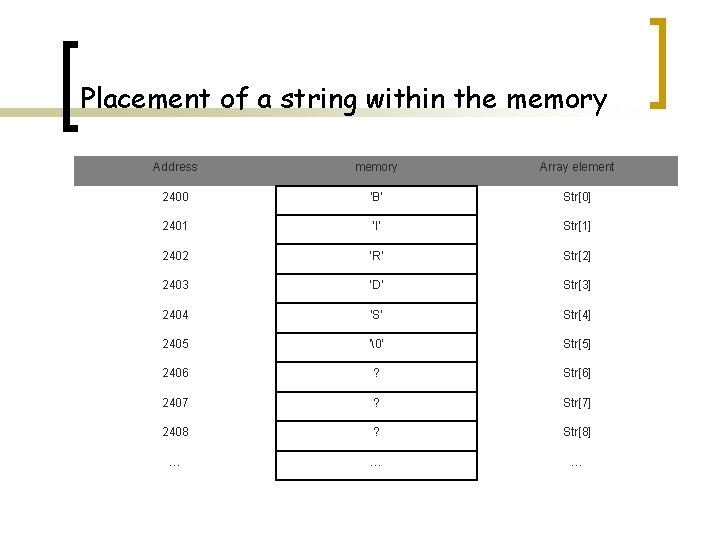Placement of a string within the memory Address memory Array element 2400 ‘B’ Str 2401 ‘I’ Str 2402 ‘R’ Str 2403 ‘D’ Str 2404 ‘S’ Str 2405 ‘’ Str 2406 ? Str 2407 ? Str 2408 ? Str … … …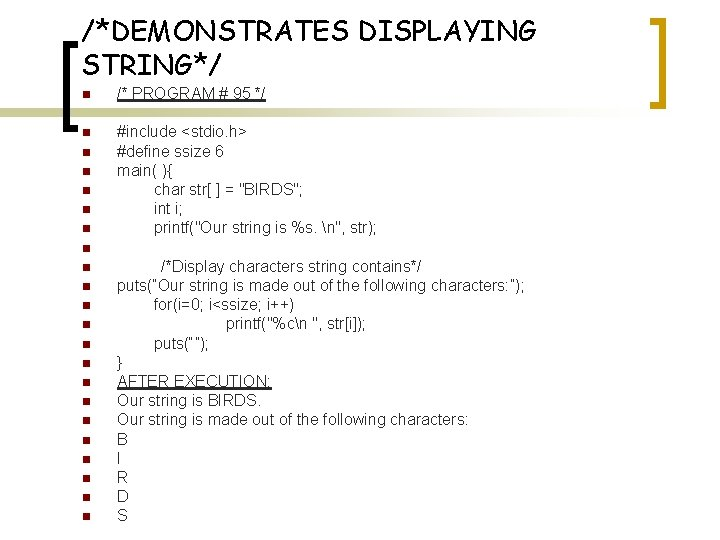/*DEMONSTRATES DISPLAYING STRING*/ n /* PROGRAM # 95 */ n #include <stdio. h> #define ssize 6 main( ){ char str[ ] = "BIRDS"; int i; printf("Our string is %s. n", str); n n n n n /*Display characters string contains*/ puts(“Our string is made out of the following characters: ”); for(i=0; i<ssize; i++) printf("%cn ", str[i]); puts(“”); } AFTER EXECUTION: Our string is BIRDS. Our string is made out of the following characters: B I R D S